# Revision Notes For CBSE Class 10 Maths Chapter 14 Statistics

In chapter 14 students will be extending the study of three statistical measures namely mode, median and mean from ungrouped data to grouped data. The chapter also discusses the concept of cumulative frequency and its distribution, drawing of cumulative frequency curves, etc. While it is important to get a good understanding of the concepts, the CBSE revision notes for class 10 maths chapter 14 – statistics provided here will help students do just that. These notes offer solid and sound knowledge of all the topics and in the process give the necessary guidance to learn the important formulas and their application in finding the right solution for the sums. The notes are the premier study materials which students can utilize and prepare well for the exams.

Students can examine the class 10 maths chapter 14 CBSE revision notes PDF below.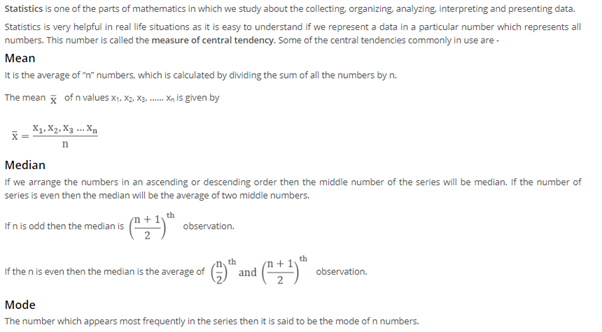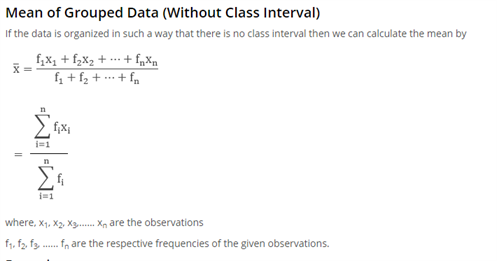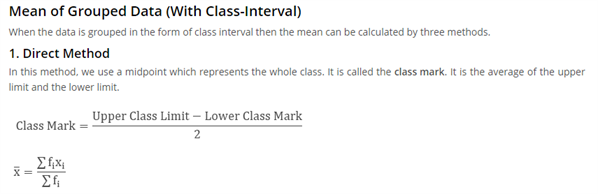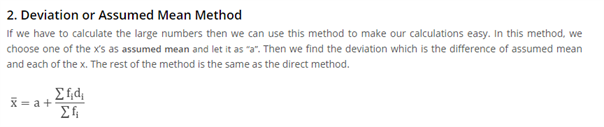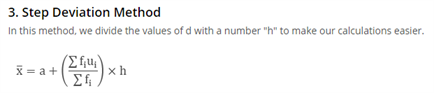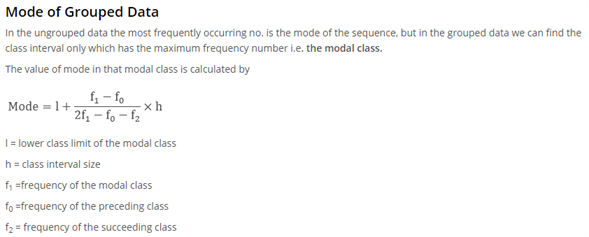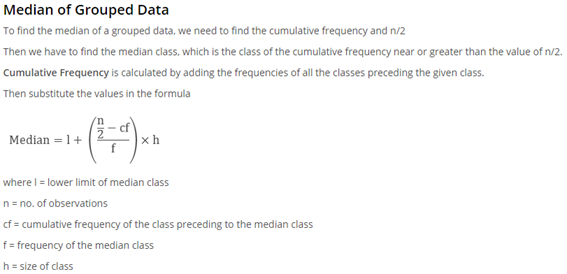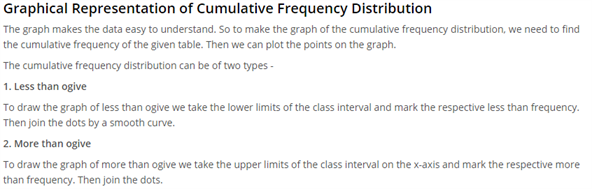Access CBSE Class 10 Maths Sample Papers Here.

Access NCERT Class 10 Maths Book Here.